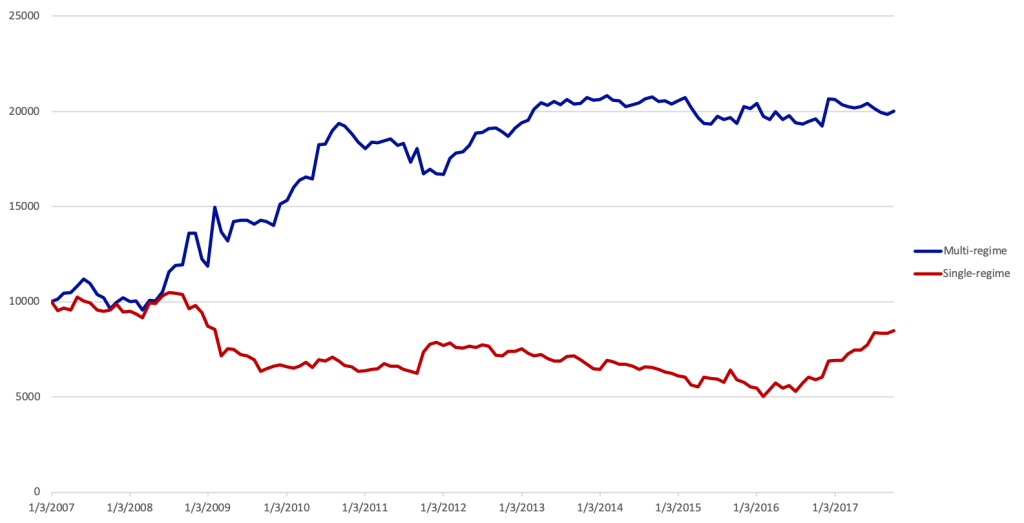## Overview

• Identification of Current Regime
• MCMC simulated over a given period of time (e.g. one month)
• Expected Return of each asset
• Monte-Carlo simulation⇒VaR of any virtual portfolio (Gaussian mixture)
• Maximization of Expected Return / VaR
• Rebalancing based on dynamic criterion (profit taking) and/or signal strength
• Benchmark: Same with 1 regime = Markowitz

## Spectral Embedding and Clustering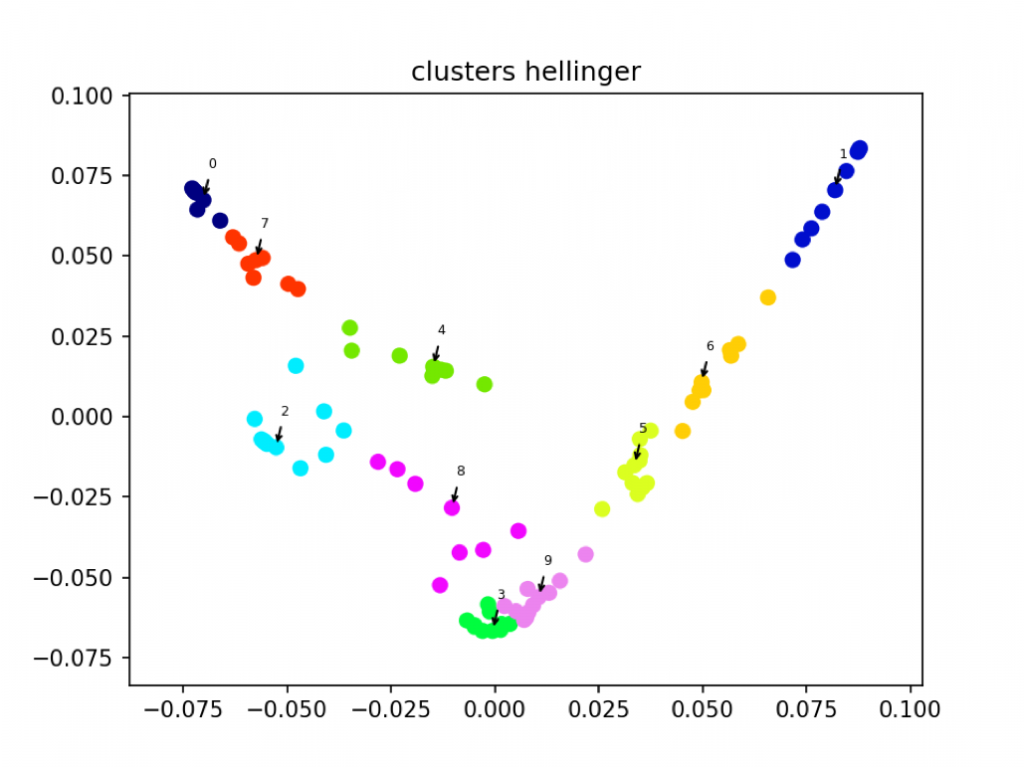## Clustering by Tree Algorithm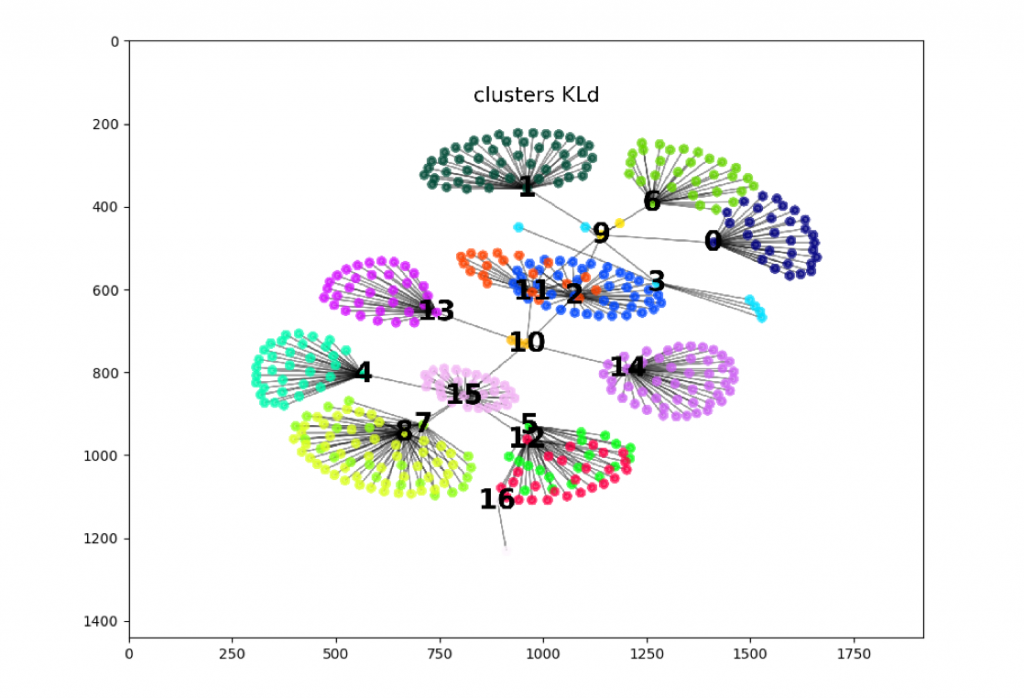## Regime Sequence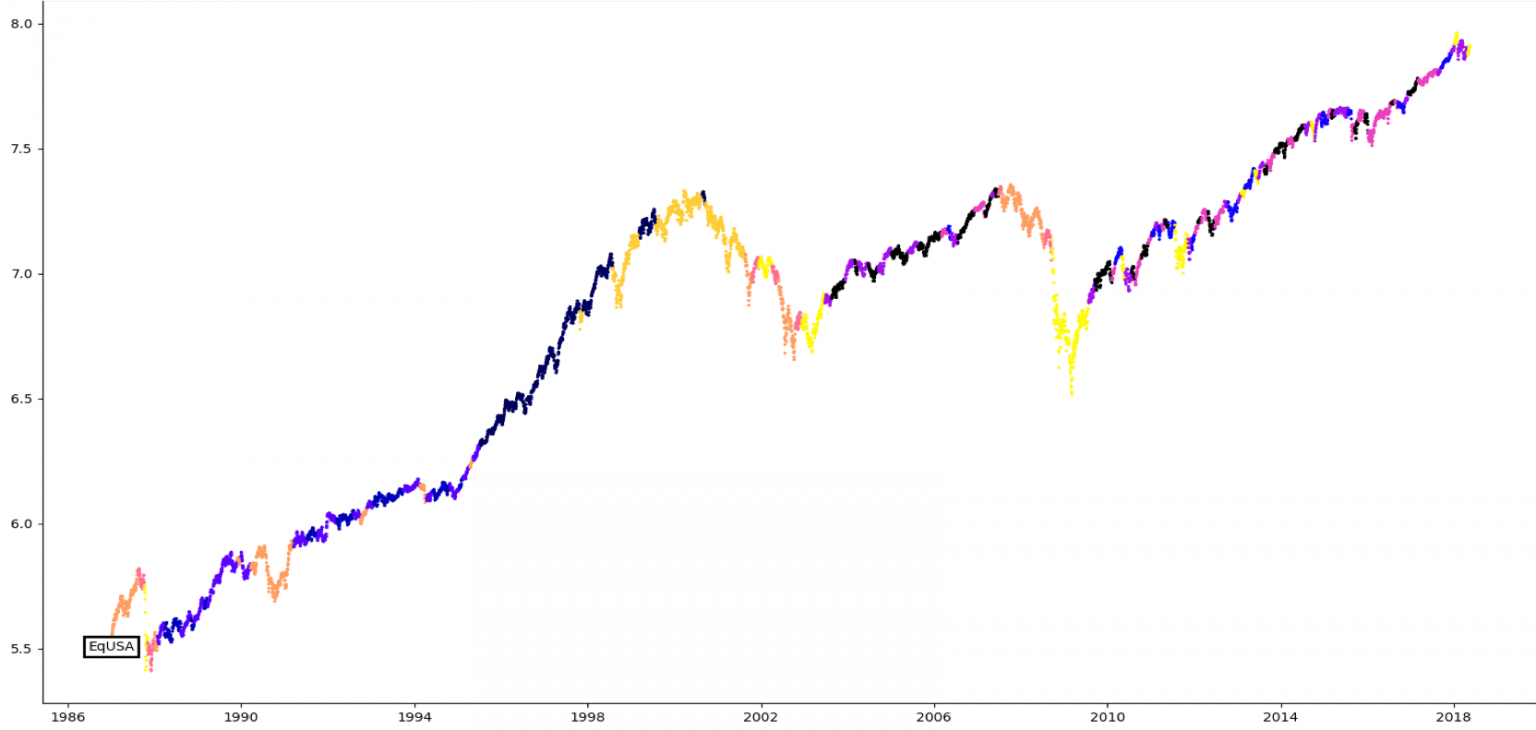• Assume that Y1Ym have mixed joint distribution

$$\ P = \pi_1P_1+…+\pi_qP_q$$ with $$\pi_1>…>\pi_q$$

• Fat tails can be measured as the ratio of risk under P1 risk under other regimes.
• An optimizer that only accounts for some regimes will reduce the risk under those regimes, but increase the risk under other regimes, hence increasing fat-tailedness

⇒Regime changes have an aggravated impact on portfolio risk

Dynamics in the Market Variable Space

• Finite Number of Regimes R1,…,Rm
• Covariance Matrix Gk
• Drift Vector mk
• Rk ~ N(mk,Gk)
• Transition Probability Matrix
• P(t,t+1) = (phk)
• phk = Probability of transitioning from Rh to Rk
• ∀h, $$\Sigma^m_{k=1}p_{hk}=1$$
• P(t,t+n) = Pn
•
•
• Euler Scheme:
• Discretized Time t0,…,tn
• Pick new regime R(ti+1) according to P applied to current regime R(ti)
• Simulate Market Evolution following R(ti+1)
• Gaussian Mixture ⇒ “Fat Tails”
• Asymptotically Gaussian
• $$\mu_∞=\Sigma^m_{k=1}\pi_k\mu_k$$
• $$\Gamma_∞=\Sigma^m_{k=1}\pi_k\Gamma_k$$
• P’ $$\pi=\pi$$, $$\pi=(\pi_1,…,\pi_m)$$
1. Determine Breakpoints($${t_1,…,t_n}$$) and “homogenous” periods $$J_k=[t_{k-1},t_k]$$
2. Calibrate a Gaussian distribution$$N(\mu_k,\Gamma_k)$$ over consecutive periods $$J_k$$
3. Clusterize the set of $$\Phi=(\mu_k,\Gamma_k)$$, using some information distance (K-L, Tsallis, Hellinger…)
4. Issue: these distributions lie in a high dimensional space n(n + 3)/2⇒No recurrence
1. Reduce dimension by describing the market with fewer indices
2. Project $$\Phi_k$$ onto a lower dimensional space, approximately preserving distances, using Spectral Embedding
• Common practice: focus only on volatility
3. The projection now has some recurrence.
5. Estimate Transition Probabilities
1. Baum-Welch algorithm: too imprecise
2. SVM or EM provide more accurate results
3. Depends on time spent within a regime
6. Crisis Prediction: Mild regime that is likely to transit to a wild one

## Multi-Regime Simulation vs. Observed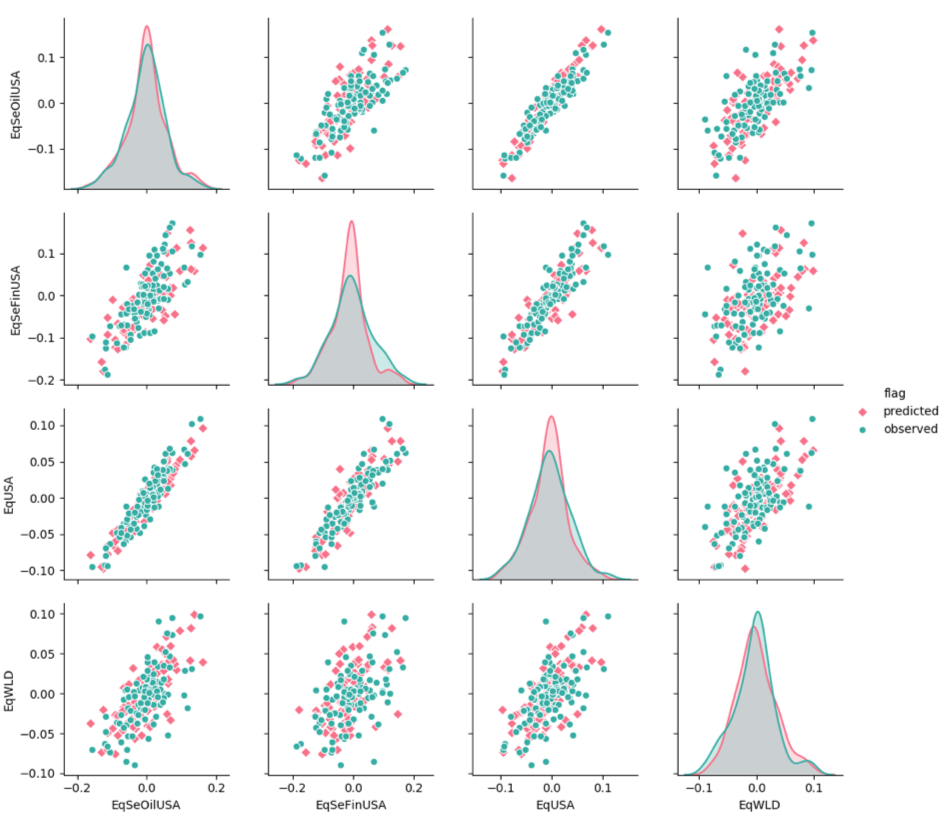Pink: Multi-Guassian Simulation (MCMC)

Green: Actual Returns

Joint distribution of 4 risk factors:

SP500

SP Sector Financials

SP Sector Oil Companies

MSCI World

## Simulation on US Market